# Trapezium Rule – approximating integrals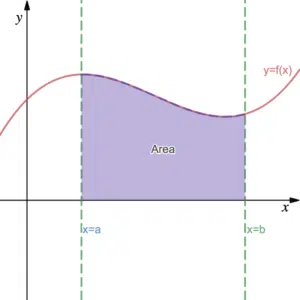Throughout this page we use methods such as the trapezium rule to approximate the area beneath a curve. This serves as an alternative to definite integrals and we will see below that the approximations can be overestimates or underestimates. Recall that the expression $\int_a^b f(x)dx$ finds the area between the curve of $f(x)$, the $x$-axis and the limits $x=a$ and $x=b$ (see definite integrals). Up until now, we found integrals using integration, that is, we find the area analytically (using algebra etc). However, if we cannot integrate $f(x)$ like this we must adopt a numerical method. For example, we could fit rectangles over the area and sum their individual areas.

## Approximating Integrals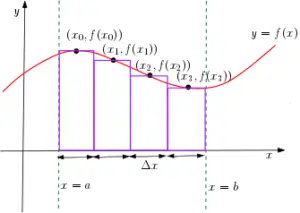In this diagram, we use 4 rectangles. Each of these rectangles has a width of $\Delta x$ (meaning change in $x$) which we can find from $\frac{b-a}{4}$ when the full width is $b-a$ and there are 4 rectangles spanning it. The height of each rectangle is dependent on the $y$-coordinate of the point on the curve which is at the centre of the top of each rectangle. Hence, the area of the $i$th triangle is $f(x_i)\delta x$. It follows that we can approximate the area in this diagram as $\sum_{i=1}^4 f(x_i)\Delta x$ (see sigma notation). The more rectangles we use the better the approximation to the integral. In general, if the number of rectangles we use is $n$ then:

$\int_a^b f(x)dx=\sum_{i=1}^nf(x_i)\Delta x$

In which case, $\Delta x=\frac{b-a}{n}$ and can be thought of the discrete version of $dx$. Similarly, we can think of $dx$ as the continuous infinitesimal version of $\Delta x$ and $\int$ as the continuous infinitesimal version of $\sum$. So, for large finite $n$, we can say $\int_a^bf(x)dx\approx \sum_{i=1}^n f(x_i)\Delta x$. However, if we take $n\rightarrow\infty$, then we can say $\int_a^b f(x) dx=\lim_{n\rightarrow\infty} \sum_{i=1}^n f(x_i)\Delta x$ where $\Delta x=\frac{b-a}{n}$.

## Trapezium Rule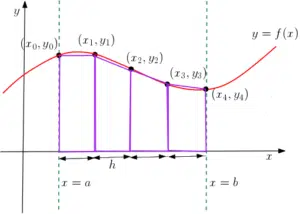In this diagram, instead of fitting 4 rectangles, we use 4 trapezia to approximate the area. In general, this is a more accurate approximation to the area. Conventionally, we use $h=\frac{b-a}{4}$ (instead of $\Delta x$) to describe the width of each trapezium. We also use $y$-coordinates to describe heights instead of the function notation. Notice that we require 5 points instead of 4 since we need the lengths of the parallel sides when computing the area of a trapezium. The area of each trapezium is ‘half the sum of the parallel sides multiplied by the distance between them‘. Hence, the area of the second trapezium here, for example, is $\frac{(y_1+y_2)h}{2}$. It follows that we can approximate the full area with the trapezium rule as:

$\int_a^b y \,dx\approx \frac{(y_0+y_1)h}{2}+\frac{(y_1+y_2)h}{2}+\frac{(y_2+y_3)h}{2}+\frac{(y_3+y_4)h}{2}=\frac{h}{2}\left\lbrace y_0+2\left(y_1+y_2+y_3\right)+y_4\right\rbrace$

where $h=\frac{b-a}{4}$. Note that,  in general, the trapezium rule for $n$ trapezia is given by

$\int_a^b y \,dx\approx \frac{(y_0+y_1)h}{2}+\frac{(y_1+y_2)h}{2}+…+\frac{(y_{n-1}+y_n)h}{2}=\frac{1}{2}h\left\lbrace y_0+y_n+2\left(y_1+y_2+…+y_{n-1}\right)\right\rbrace$

where $h=\frac{b-a}{n}$. This formula is given in the Edexcel Formula Booklet. See Examples 1 and 2 for a demonstration of the trapezium rule in use.

### Overestimate or Underestimate?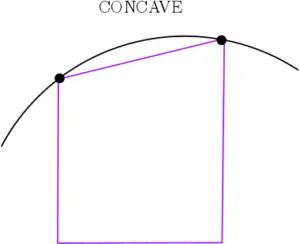The trapezium rule will only ever give the exact area if the graph has the same shape as the trapezia. In general, the trapezium rule will give an overestimate or an overestimate. Which one of these it is will depend on the shape of the curve.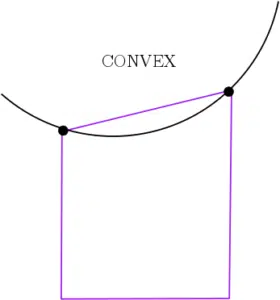Recall the difference between a concave and a convex function. The trapezium rule applied to parts of the graph where it is concave will give an underestimate. Conversely, the trapezium rule applied to parts of the graph where it is convex will give an overestimate.

It is harder to tell what kind of estimate we get using rectangles instead. This is because, the rectangle don’t tend to follow the line of the graph as closely as the trapezia do.

## Examples using the Trapezium Rule

The following shows the graph of the function $f(x)=2-\cos^2(x)$ and the dashed line $x=1$: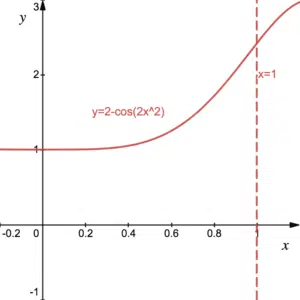1. Complete the following table of values, giving 3 decimal places where appropriate:

 x 0 0.2 0.4 0.6 0.8 1 f(x) 1 2.416

2. Using the trapezium rule with 5 trapezia, estimate the area bounded by the curve, the $x$-axis, the $y$-axis and the line $x=1$.
3. Is the estimate an overestimate or an underestimate? Explain why increasing the number of trapezia improves the approximation.

The following shows the graph of $y=5\sqrt{x}+3$ and the dashed line $x=1$: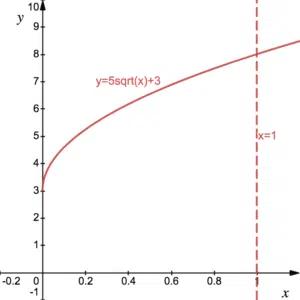1. Use the trapezium rule with 5 trapezia to approximate the area between the curve, the $x$-axis, the $y$-axis and the line $x=1$. Is this approximation an overestimate or an underestimate?
2. Use integration to find the exact area.
3. What is the percentage error in the approximation?